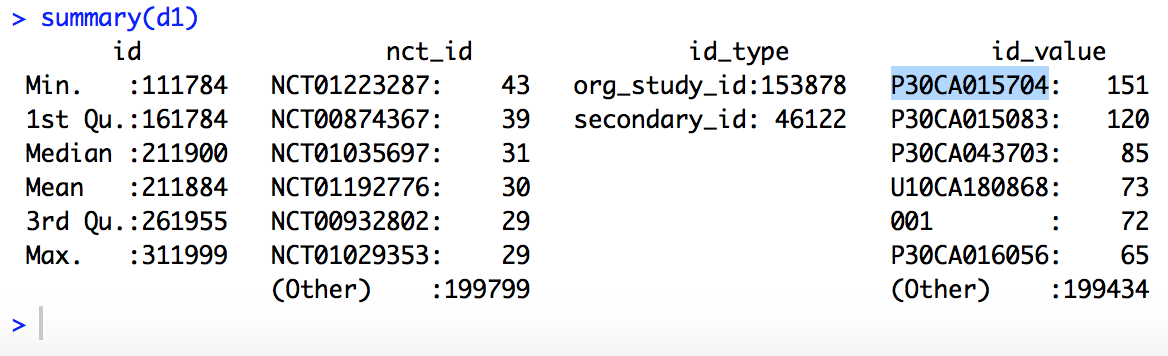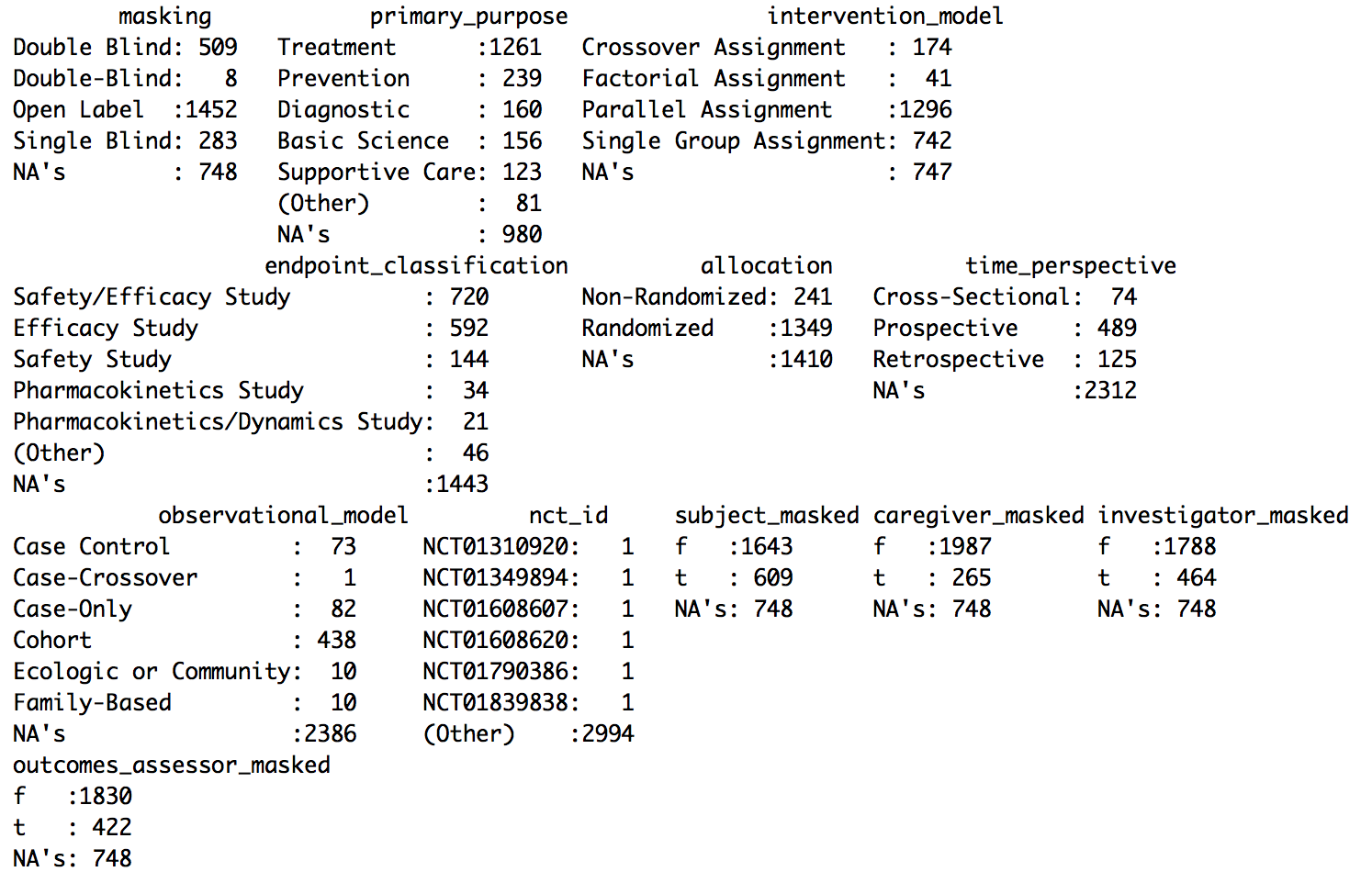## Tips for Analyzing Data in Pipe-Delimited Files Using R

The pipe-delimited text files can be read using the read.table() function in base R. R is powerful and can provide a lot of useful information with very little effort (as demonstrated in the instructions below). Note: These instructions were tested using R 3.1.2 installed on a Linux platform.

1

If R (and RStudio) not already installed on your local machine, you may install from the following links:

2

### Start by Reading a Small File

Assuming you have installed/launched R, and have downloaded/unzipped a set of AACT pipe-delimited files...

When getting started, first try reading a smaller file (e.g., ‘id_information.txt’). This may help troubleshoot minor issues. Example R code:

```
sep = "|",
na.strings = "",
comment.char = "",
quote = "\"",
fill = FALSE,
nrows = 200000)

summary(d1)    # print a summary of dataframe d1

```

R should respond with something like:Notes:

• R assumes the file ‘id_information.txt’ is in the current working directory. Substitute appropriate path and file information as needed.
• Decreasing nrows value may improve processing time, but also decreases the sample size. Make sure it's set large enough to be meaningful. Refer to the AACT Data Dictionary for information about expected row counts. The ‘sanity_checks.txt’ file also provides information about number of records, frequently encountered variable values, and maximum variable length or each data file.
• The quote = "\"" argument enables quoting of character variables using the " character. This is needed to avoid reading embedded | within a string as a field delimiter.
3

Once you have successfully read a small file, try reading the file ‘designs.txt’. This file is larger, but still contains just simple structured data elements (no free text). Reading this file may help test memory requirements on your system, and is a good file on which to develop strategies for reading larger files. (To try this out, replace id_information.txt with designs.txt in the previous code sample and rerun in R.)

R should respond with something a bit more interesting like:3.a

### Read Small Number of Rows First to Determine Memory Requirements

Example code:

```
na.strings = "",
stringsAsFactors = FALSE,
comment.char = "",
quote = "\"",
fill = FALSE,
nrows = 1000)

```

Notes:

• Specifying stringsAsFactors=FALSE prevents character variables from being converted into factors.
• Because nrows=1000 is specified, only the first 1000 records will be read. This is too little, but sufficient for evaluating memory requirements.
3.b

### Determine Memory Requirements

The following code can be used to estimate the approximate memory needed to read the full dataset

```
sz <- object.size(d0)   #  determine approximate memory used to store d0
#  multiply by (nrows for full data set / 1000)
#  to estimate total memory needed to store the full data
print( sz * 250000 / 1000, units="Mb")
#  Estimate that ~50 Mb of memory will be needed.
#  This is fine since my system has > 4 Gb of RAM.

```

3.c

```
sep = "|",
na.strings = "",
colClasses = c("integer", rep("character",14) ),
comment.char = "",
quote = "\"",
fill = FALSE,
nrows = 250000)    # a bit larger than needed

```

3.d

### If Memory Constraints Prevent Access - Try Reading Subset That Contains Studies of Interest

Example 1: Read rows 1000 to 1999 in the data file:

```
skip = 999,            # skip first 999 records
nrows = 1000,          # read the next 1000 records
col.names = names(d0), # use column names determined from d0
na.strings = "",
sep = "|",
comment.char = "",
quote = "\"",
fill = FALSE,
nrows = 250000)    # a bit larger than needed

```

To identify row number(s) containing string "NCT00000558"...

```
 222688

```

The above reveals that NCT00000558 occurs in line 222,688 of the designs.txt file.

```
# Read just the desired lines with read.table using skip and nrows
skip = 214443,         # skip first 214443 records
nrows = 1,                # read 1 record
col.names = names(d0), # use column names determined from d0
na.strings = "",
sep = "|",
colClasses = c("integer", rep("character",14) ),
comment.char = "",
quote = "\"",
fill = FALSE)

```

4

### Investigate Content of Other Files

Once you have successfully read the two files mentioned above, you are ready to try reading some other files. The file ‘studies.txt’ contains one record per study (NCT_ID is the unique identifier), and contains many of the study protocol data elements.

The following code reads the first 1000 records from the file ‘studies.txt’.

```
sep =  "|",
na.strings =  "",
stringsAsFactors =  FALSE,
comment.char =  "",
quote =  "\"",
fill =  FALSE,
nrows =  1000)

```

Check memory requirements, assuming ~ 250000 rows: sz <- object.size(d0) print( sz * 250000 / 1000, units="Gb")

```
#  Specify appropriate variable types based on d0 and Data Dictionary

Classes = c(
"character",
rep("Date",3),
rep("character",6),
"integer",
rep("character",6),
rep("integer",2),
rep("character",11),
"Date",
rep("character",6)
)

```

```
#  read full dataset of studies